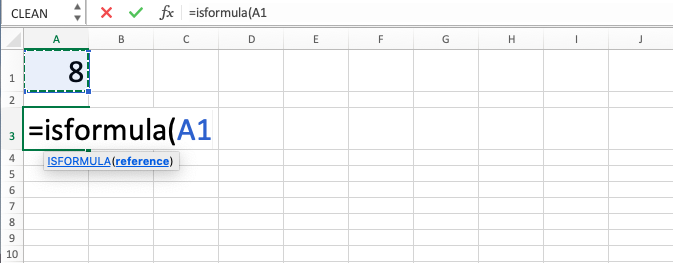ISFORMULA Excel Formula - Compute Expert

# ISFORMULA Excel Formula

In this tutorial, you will learn the use of the ISFORMULA excel formula. ISFORMULA function in excel is useful to check whether a cell contains a function or not.

## Why do We Need to Learn ISFORMULA Function in Excel?

In the data processing on a spreadsheet, sometimes we need to know whether a cell contains a formula or not. This is needed for various needs such as analyze the errors that we have in the spreadsheet or identify material for further analysis needs. If we use a manual way to see one by one the contents of our cells, then it will be very troublesome especially if there are many cells that we need to check to fulfill our needs.

As an example of this need, imagine if we find many errors in the data processing that we do on the spreadsheet. The errors can be caused by various things such as external data or formulas which are wrong in their implementation. If we know which cells contain formulas, then we might be able to do the fixes according to the appropriate needs. For that, we need to do an identification process first to check whether a cell contains a formula or not. However, if there are many cells to be identified then it will be troublesome and there will be a lot of time that we need for the identification process.

To help make the identification process much faster, you can use the ISFORMULA excel formula. ISFORMULA function in excel is useful to evaluate a cell whether it contains a function or not and give a TRUE or FALSE logic value based on the evaluation result. It is very useful to be learned and used if you sometimes need this kind of identification process in your data processing process on the spreadsheet.

## What is ISFORMULA Excel Formula?

ISFORMULA excel formula is a function with the usage objective to evaluate the content of a cell whether it is a formula or not and gives TRUE or FALSE based on the evaluation result. This function is one of the IS functions on the spreadsheet besides the other ones such as ISERROR and ISNUMBER.

ISFORMULA excel formula is sometimes used with IF formula because IF can do further processing from the TRUE or FALSE which is resulted.

Generally, the input of this function can be explained as follows:

=ISFORMULA(value)

Note:
value = cell coordinate that you want to check the content of

## How to Use ISFORMULA Function in Excel?

The following will explain how to write the ISFORMULA function in excel. It only needs one input which is the cell coordinate to be checked whether it contains a function or not.

## Using ISFORMULA Excel Formula

1. Type equal sign ( = ) in the cell where you want to put the result in2. Type ISFORMULA (can be with large and small letters) and open bracket sign after =3. Input the cell coordinate that you want to check after open bracket sign4. Type close bracket sign5. Press Enter
6. The process is done!## Exercise

After you have learned how to use ISFORMULA function in excel, it is time to deepen your understanding by doing the following exercise.

### Instructions

Fill your answers on the gray-colored cells in the appropriate row number
1. Is the data on the left a formula? Use TRUE or FALSE in your answer!
2. Is the data on the left a formula? Use “V” if yes or “X” if not in your answer! Use the IF solution to help you answer the question!
3. Is the data on the left a formula? Add 80 on the number if yes and turn it into 0 if not! Use IF again to help you answer this question!

ISFORMULA function in excel can be used from the 2013 version of this software tool.

Get updated excel info from Compute Expert by registering your email. It's free!